Livio/ May 30, 2019/ Python/ 0 comments

Linear Regression in Python

In this post I wanted to show how to write from scratch a linear regression class in Python and then how to use it to make predictions. Let’s start!

What is Linear Regression

Linear regression is a technique of modelling a linear relationship between a dependent variable and independent variables. When the independent variable is just one, it is known as Simple Linear Regression, otherwise it is known as Multiple Linear Regression. The class we will build will deal with both. Linear Regression makes certain assumptions, such as the lack of multicollinearity which happens when two or more predictor variables are perfectly correlated.

Given a vector of dependent variables y (M x 1) and a matrix of independent (predictor) variables X (M x N), linear regression assumes that their relationship is linear and can be written as: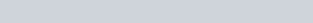Our goal will be to find the values of B that minimize the sum of squared errors: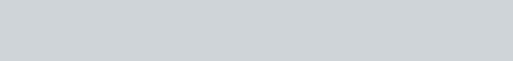where an error is defined as the difference between the predicted value: b0 + b1X1 + b2X2 and the observed value Y.

In order to do that, we will use a technique called Gradient Descent.

Gradient descent is an iterative algorithm which finds the minimum of a function. In our case, the function we are trying to minimize is the Cost function shown above. To make it easy to understand, imagine we’re dealing with a Simple Linear Regression scenario. This means that relationship is defined by: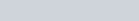And the Cost function to minimize is: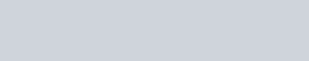In order to find the minimum, we first need to compute the derivative of the cost function with respect to B0, which is: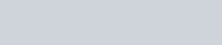then we start by assigning a random value to B0, we calculate the derivative and we decrease or increase the value of B0 by the calculated derivative. If the derivative is positive, it means the slope is positive therefore we need to decrease the value of B0, and need to do the contrary if the value of the derivative is negative. The new value is: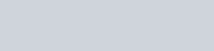where alpha is a learning rate. We need to repeat this process iteratively until the value of the derivative is zero (function has reached its minimum).

But, as you have seen above, we also need to estimate the value of B1, therefore we also need to calculate the derivative with respect to B1 and repeat the same process. The vector containing the partial derivatives of a function with respect to different parameters is known as gradient. And so our gradient is:Our class will then need to calculate the derivative of the cost function with respect to each coefficient, then calculate the new coefficients and repeat this process until the minimum has been reached.

# Standard score

In order for our gradient descent algorithm to be more efficient, we will standardize our Xs matrix and our Ys matrix. Standard scores are calculated easily by subtracting the mean and divide by the standard deviation. When we standardize, our equation becomes: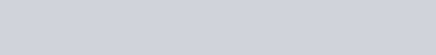The coefficients calculated this way are known as standardized coefficients.
After we have calculated them, if we wish to convert them to their non standardized version, we need to follow the following steps:So our intercept (b0) and our coefficients will be:Building the class in Python
Below we will build the Linear Regression class from scratch. The class is making use of Numpy, a must-have library for scientific computation in Python.
__init__ method:
The init method contains just one argument, standardize, which determines whether to return standardized or de-standardized coefficients:
predict method:
the predict is used to output the predictions based on matrix ‘predictors’ and a coefficients vector:

_errors method:

The _errors method is used to output a vector of the differences between the observed values (ys) and the predicted values:

_sum_of_errors method:

The _sum_of_errors method returns the sum of the vector of _errors:

_sum_of_squared_errors method:

The _sum_of_errors method returns the sum of the squared elements of _errors:

_derivatives method:

The _derivatives method will return a vector of the derivatives corresponding to the jth attribute:

_new_coefficients method:

This method will return a vector of new coefficients calculated:

standardization methods:

The below methods are used to standardize and de-standardize the observed values (ys), the predictors variables(xs) and the coefficients:

fit method:

The fit method is the one used to find the best values for the coefficients:

Other properties:

Below are three more properties available within the class:

Below you will find the full class code:

Normal Equation

Normal equation is an alternative to gradient descend to find the coefficients which minimize the cost function. We need to think about this in terms of matrix calculus where the cost function becomes:where X is a (m X n) matrix, theta is a (n X 1) matrix and Y is a (m x 1) matrix. If we calculate the derivative and solve for theta we get a vector of coefficients which minimize this cost function: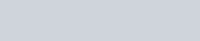In this case, the fit method of my previous post, which is used to find the coefficients that minimize the cost function, becomes much simpler:

It is worth nothing that this method will only work if the below matrix is invertible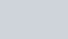Using the class we have created

Simple Linear Regression

The data set we will use for simple linear regression can be downloaded at this link. It is a simple data set containing two column with car insurance data. Column 1 contains our predictor variables (number of claims for a specific region) and column 2 contains our Ys (total payment for all the claims in thousands)

or in a Jupyter notebook: